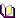## Boring logs

The display of soil properties C and Fhi is optional. Also it is optional to display many borings together (Figure B-49).

Soil colors according to German Standard DIN 4023 is considered automatically, if desired, in the drawing of boring logs (Figure B-50).Figure B-49 Many borings togetherFigure B-50 Boring log with soil colors according to German Standard DIN 4023

## Language of the help

You can define the language of the help system used in ELPLA applications (Figure B-43 to B-46). The three languages are English, German and Arabic.Figure B-43 Menu "Help language settings"Figure B-44 Help language in GermanFigure B-45 Help language in EnglishFigure B-46 Help language in Arabic

## Determining stresses, strains and displacements in soil

You can determine the stresses, strains and displacements in soil under the foundation in a net in z-direction (Figures B-35 to B-38).

ELPLA can display results of stresses, strains and displacements in different forms such as:

 - Soil deformation as deformed mesh (Figure B-39). - Soil deformation as vectors (Figure B-41). - Principal soil stresses as streaks (Figure B-41). - Principal soil strains as streaks (Figure B-42).Figure B-35 Menu "Net of soil elements in z-direction"Figure B-36 Z-Stresses Sigma_zFigure B-37 Z-Strains Epsilon_zFigure B-38 Z-Displacements wFigure B-39 Soil deformation as deformed meshFigure B-40 Soil deformation as vectorsFigure (B-9) Principal soil stresses as streaksFigure B-42 Principal soil strains as streaks

## Analysis of grid

Besides the three different analyses available in the program ELPLA to analyze foundations and floor slabs, a new analysis "Analysis of grid" is added (Figures B-33 and B-34).Figure B-34 Beam-MomentsEnhancements in ELPLA 8.1